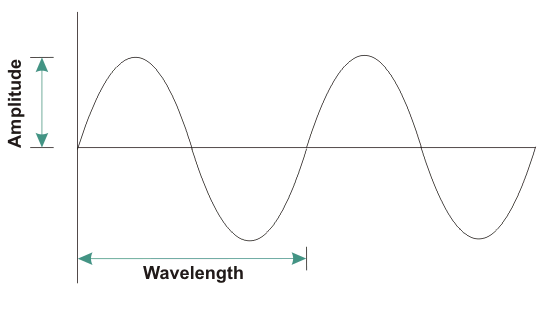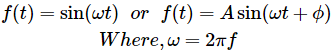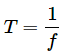# Sinusoidal Wave Signal

To understand what is Sine wave or Sinusoidal Wave Signal, first let us try to understand what a Signal is.

## What is a Signal?

There are different measurable quantities in the world surrounding us. Some quantities are constant like acceleration due to gravity, speed of light, velocity of sound in air. Some are time-varying like AC voltage, Pressure, Temperature. It means they change their value as time passes on. Signal simply means the value of any quantity taken over a period of time. Signals are usually time varying in nature. Generally a graph is plotted between values at different time instants. This is called graphical representation of signal.

## What is Sine Wave or Sinusoidal Wave Signal?Sine Wave or Sinusoidal Wave Signal is a special type of signal. It is given by the functionWhen Sine wave starts from zero and covers positive values, reaches zero; and again covers negative values, reaches zero, it is said to have completed one cycle or single cycle.
The upper part of sine wave is called positive cycle and the lower part is called negative cycle in a single cycle.
For different values of time, the Signal gives the values of quantity at that time. Therefore Signal is a function of time. It is therefore written asf (t). The Maximum value of the Sinusoidal Signal is also called its amplitude (A). Here ω is called Angular Frequency of Signal and f is the Frequency of Signal. ∅ Is called Phase difference.
Frequency is measured in Hertz (Hz). It shows number of cycles of signal that took place in a second. Large ω or large f value indicates that the signal completes more oscillations (i.e., going from positive values to negative values) in less amount of time. Hence the Signal is more Oscillatory in nature.

Sinusoidal signal need not start at zero. It may start after certain duration of time. This is time after which Sinusoidal Signal starts is indicated with the help of phase difference (∅). It is measured in Radians.
Periodic signals are those which repeat their pattern after certain amount of time. This time after which pattern is repeated is called time period (T) of Periodic Signal. It is inverse of frequency of Signal.Sinusoidal signal is a periodic signal, because the pattern keeps on repeating after one Wavelength as shown in the Figure above.
All the power signals in our home, office and industries are AC sinusoidal signals. The frequency (f) in India and British countries is 50 Hz and in American countries it is 60 Hz.

## Why is Sinusoidal Wave Signal so Important?

Sinusoidal signals are important in both electrical and electronic engineering domains.
According to Fourier Series Theory, any signal (Periodic Signal) can be written in terms of only sine and cosine Signals of different frequencies. Therefore a complex signal can be broken-down into simple sine and cosine signals and mathematical analysis becomes easy. Hence it is widely used in electrical and electronic analysis.
Also, in transformers the output voltage is time derivative of magnetic flux. Magnetic flux is itself time derivative of input voltage. But we want same voltage signal both at input and output. The only functions that satisfy this condition are sine and cosine functions. As sine signal starts from zero value, it is preferred. Therefore majority of power systems in the world today are using sinusoidal AC voltage. All the household equipment also work on Sinusoidal AC voltage.

Want To Learn Faster? 🎓
Get electrical articles delivered to your inbox every week.
No credit card required—it’s 100% free.